# What is solubility and dissociation

The solubility of a substance indicates whether and to what extent a pure substance can be dissolved in a solvent. It describes the property of a substance to mix with the solvent with homogeneous distribution (as atoms, molecules or ions). Most of the time the solvent is a liquid. But there are also solid solutions, such as alloys, glasses, ceramic materials and doped semiconductors. In the case of the solution of gases in liquids, the term solubility refers to a coefficient which indicates the amount of gas dissolved in the liquid in diffusion equilibrium with the gas space in relation to the pressure of the gas.

One distinguishes the

• qualitative solubility (is the substance soluble in a certain solvent?) and the
• quantitative solubility (what amount of substance can be dissolved in the unit volume of a certain solvent?).

### Qualitative solubility

Today we know that at temperatures above absolute zero there must always be a certain solubility for every substance in every other substance for thermodynamic reasons (entropy) alone. The increasingly precise analysis methods confirm this. Strictly speaking, therefore, a distinction between soluble and insoluble cannot be made. It is therefore a question of semiquantitative determinations such as poorly soluble, limited soluble or unlimited soluble.

The liquids in which a solid is readily soluble depends on the molecular properties of the substance and the liquid. Salt-like substances (ionic compounds) are almost only soluble in polar solvents such as water or hydrogen fluoride (HF). Many lipophilic, e.g. B. waxy substances, on the other hand, are only appreciably soluble in organic solvents such as gasoline (an "apolar" solvent). In this context, “polar” means that the molecules of the solvent have a dipole moment and therefore interact with charged (ions) or polar molecules of the substance to be dissolved, but without a reaction occurring. The polarity of solvents is scalable. Different polarities and thus different solubilities are used extensively in chromatography processes.

Many substances, such as ethanol, can be mixed well with both water and non-polar solvents. Acetone can be mixed with almost any liquid.

### Quantitative solubility

The solubility of a substance in a solvent is not limited in all cases. Sulfuric acid can be mixed with water in any ratio.

When mixing phenol with water, on the other hand, there are two areas: a solution of phenol in water and a solution of water in phenol. In between, an area of ​​“forbidden” mixing ratios is left out, which leads to the formation of a stratification of two liquid phases.

The quantitative solubility or Solubility limit For systems with limited solubility, indicates the maximum concentration of one substance in the other in which the mixture is still single-phase under equilibrium conditions. The solubility limit is temperature dependent. If the solubility limit is exceeded, a second phase is eliminated. If the necessary activation energy or diffusion for the separation of the second phase is missing, the mixture remains single-phase in a metastable, supersaturated state even above the solubility limit. In the case of salts, the solubility follows from the Solubility product. If none of the ions involved is also available from another source, the solubility of the salt in question can be calculated: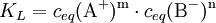The solubility of the salt AmB.n: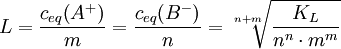In mixed solutions, as in z. B. natural waters, it regularly happens that the concentrations of anions and cations do not match stoichiometrically in pairs, as it would correspond to the solution of individual salts. An important example of this is dissolved lime, which dissolves as calcium hydrogen carbonate through dissolved carbon dioxide and makes the essential contribution to water hardness. Due to the dissociation equilibrium of the carbonic acid, with the constantly changing carbon dioxide content of the water (e.g. through breathing and photosynthesis of the aquatic organisms), the concentrations of the carbonate and hydrogen carbonate anions are shifted, while the concentration of the calcium cations initially remains unaffected . In this case, the product of the by no means equivalent concentrations of calcium and carbonate ions decides whether and to what extent calcium carbonate will precipitate (e.g. as scale or sea chalk) because the solubility product is exceeded.

The quantitative solubility, like the concentration of solutions in general, is given in different units:

• g / l solution
• g / 100 g solution (mass percent)
• ml / 100 ml solution (percent by volume,% by volume)
• g / kg solution
• mol / l solution (molarity)
• val / l solution (normality)
• mol / kg solvent (molality)

### Example for the solubility calculation of a single salt

Calculation of the solubility L. for aluminum sulfate in water, if the K is knownL.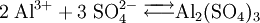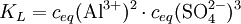This means that every mole of aluminum sulfate in the solution produces 2 moles of aluminum and 3 moles of sulfate ions. The following relationship between the reaction constant K also appliesL. and the solubility L: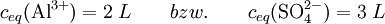The factors in front of L are explained by the stoichiometric factor.
This is now put into the equation for KL. one, it then follows: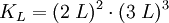in order to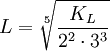The numerical values ​​for the solubility products are obtained from the standard free enthalpies (see Gibbs energy).

### Solution of gases in liquids

At the interface between gases and liquids, gas molecules diffuse between the gas space and the solution space. The entry of molecules into the solution is proportional to the partial pressure of the gas and the exit is proportional to the concentration of the gas in the solution (see Henry's law). At the so-called saturation concentration there is a dynamic equilibrium between the two diffusion directions. The saturation concentration is proportional to the partial pressure in the gas space. The connecting constant of proportionality is referred to here as solubility:

Gas solubility (Gas i) = saturation concentration (i) / partial pressure (i)

As a rule, this solubility of gases in liquids decreases with increasing temperature. A deviation from the proportionality between gas pressure and equilibrium concentration is only noticeable at high pressures.

### Dissolution of metals in metals

In normal usage, solutions are usually thought of as liquid media, but there are also solid solutions (at room temperature), e.g. B. the alloys, so solutions of metals in metals. They are widely used because of their special material properties. Bronze is the oldest consciously man made alloy. Brass and the various steels are well-known examples. Jewelery gold is usually an alloy of gold with silver (yellow gold) or copper (red gold), the gold content of which is given in carats.

### See also

Category: substance property